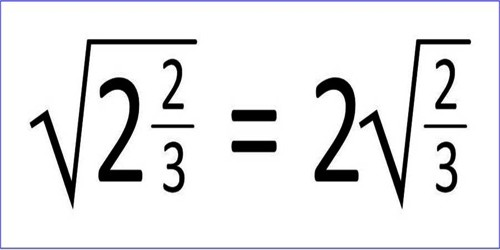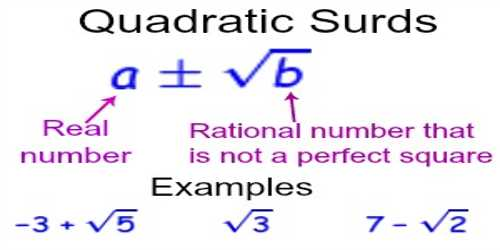Geographic Minerals

# Express of a Simple Quadratic SurdA root of a positive real quantity is called a surd if its value cannot be exactly determined. It is a number that can’t be simplified to remove a square root (or cube root etc). For example, each of the quantities √3, ∛7, ∜19, (16)^2/5 etc. is a surd.

A quadratic surd is an irrational number that is the solution to some quadratic equation with rational coefficients which is irreducible over the set of rational numbers. A number of the form (±√a), where ‘a’ is a positive rational number which is not the square of another rational number is called a pure quadratic surd. A number of the form (a ± √b), where ‘a’ is rational √b is a pure quadratic surd is sometimes called a mixed quadratic surd.Express of a Simple Quadratic Surd

We cannot express a simple quadratic surd by the following ways:

• A simple quadratic surd cannot be equal to the sum or difference of a rational quantity and a simple quadratic surd.

Suppose, let √p a given quadratic surd.

If possible, let us assume, √p = m + √n where m is a rational quantity and √n is a simple quadratic surd.

Now, √p = m + √n

Squaring both sides, we get,

p = m^2 + 2m√n + n

m^2 +2m√n + n = p

2m√n = p – m^2 – n

√m = (p – m^2 – n)/2m, which is a rational quantity.

From the above expression, we can clearly see that the value of a quadratic surd is equal to a rational quantity which is impossible.

Similarly, we can prove that √p ≠ m – √n

Therefore, the value of a simple quadratic surd cannot be equal to the sum or difference of a rational quantity and a simple quadratic surd.

• A simple quadratic surd cannot be equal to the sum or difference of two simple, unlike quadratic surds.

Suppose, let √p be a given simple quadratic surd. If possible, let us assume √p = √m + √n are two simple quadratic surds.

Now, √p = √m + √n

Squaring both sides we get,

p = m + 2√mn + n

√mn = (p – m – n)/2, which is a rational quantity.

From the above expression we can clearly see that the value of a quadratic surd is equal to a rational quantity, which is obviously impossible since √m and √n are two unlike quadratic surds, hence √m ∙ √n = √mn cannot be rational.

Similarly, our assumption cannot be correct i.e. √p = √m + √n does not hold.

Similarly, we can prove that, √p ≠ √m – √n.

Therefore, the value of a simple quadratic surd cannot be equal to the sum or difference of two simple, unlike quadratic surds.

Information Souce: